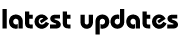Physics : Theories Of Relativity

SPECIAL AND GENERAL THEORIES OF RELATIVITY

Special theory of relativity was formulated by Einstein.

SPECIAL RELATIVITY

The special theory of relativity states that nothing can exceed the speed of light, which is the same in all inertial (nonaccelerating) time frames and that all inertial time frames are equally good for carrying out experiments. Special relativity equates space with time and matter with energy. In special relativity time and space have to be considered as unified and not as two separate things; this merged entity is known as space-time. Matter and energy are aspects of the same phenomenon, matter energy, and are linked in the famous equation: E = mc2 where E is the energy released when mass (m) is destroyed, as in a nuclear reaction, and c is the velocity of light. It indicates that when 1 kg is destroyed, 9 x 1016 joules of energy are released.

GENERAL RELATIVITY

In his general theory of relativity, Einstein considered systems accelerating with respect to each other. He concluded that the effects of acceleration and gravity were equivalent. Using the theory, he was able to explain a discrepancy in the slow rotation of the planet Mercury’s elongated orbit, which Newtonian mechanics had been unable to explain. Einstein explained the effect of a large concentrated mass, in this case the Sun, by saying it ‘warped’ the space around it. Thus space, or rather spacetime, is curved. The degree of curvature depends upon the magnitude of the mass, and so matter-energy determines the curvature of space-time.

HEISENBERG UNCERTAINTY PRINCIPLE

The Heisenberg Uncertainty Principle (1927) concerns the accuracy with which the position and momentum of a particle (such as an electron) can be determined. Thus an accurate determination (measurement) of one quantity leads to the impossibility of measuring the other accurately. As both quantities cannot be known precisely, it follows that limits are set on the accuracy of any predictions made using these measurements.

STRING THEORY

One of the problems with current models of physics is that relativity and quantum theory are, to some extent, inconsistent with each other. So far, no physicist has been able to provide a quantum theory of gravity which would combine the two fields. This search for a Grand Unified Theory (GUT) has occupied many scientists for much of this century. A recent possible theoretical model is string theory. String theory suggests that the universe is made up of very small vibrating strings. These strings are about 100 billion billion times smaller than a proton, which itself is less than a billionth of a metre in size. In addition, these strings are vibrating in ten dimensions.

The different resonances at which these strings vibrate produce the varied fundamental particles, such as quarks, leptons and electrons, that are known to exist. String theory also predicts the relativistic equations of  Einstein. Thus the theory has managed to unify several separate aspects of modern physics. However, it too is not yet complete: there are many millions of mathematical solutions to the field theory of strings, and the correct solution is still beyond grasp of  contemporary physics.My Great Web page